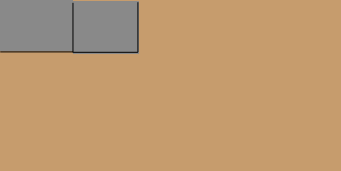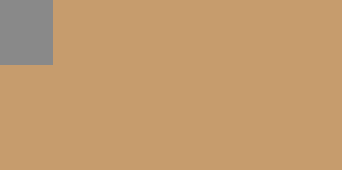SEARCH HOMEMath Central Quandaries & QueriesA question from Joe. How many cinder blocks 16x8 would i need to build a solid base for a plywood base 54 inches wide and 30 inches deep Thank youHi Joe,

Suppose you place the blocks with the long side, 16", along the long side of the plywood, 54".$\frac{54}{16} = 3.375$ so it will take a row of 4 blocks plus a little more to cover the length of the base. Each block is 8 inches wide and the base is 30 inches wide so how many rows of 4 blocks will it take to cover the base? How many blocks is that in total?

Suppose now you decide to arrange the blocks in the other direction. How many bocks will it take to make a row to cover the 54 inch length of the base?How many of these rows do you need? What is the total number of blocks?

Which arrangement requires fewer blocks?

PennyMath Central is supported by the University of Regina and The Pacific Institute for the Mathematical Sciences.